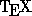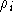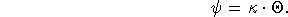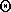Next: Beginning to Type Up: An Introduction to Using Previous: Files

# Text Modes

So now you're in the file and ready to type beautiful (and, hopefully, correct) mathematics. One thing always to keep in mind is thatcommands--i.e., words which whened'' turn into symbols or directions to do things like underline, center, etc.--are usually preceded with a backslash, \ .

Basically, the stuff you type falls into two categories: math and text., correspondingly, has two modes,'' math mode and text mode, which determine how it responds to the text and commands in your file. Text mode is the default. Most commands in text mode change the appearance and layout of the text on the page (what font it is in, for instance, and whether it is centered). In time we'll see examples of most of the useful ones.

Math mode is indicated by a dollar sign: $ . Everything between dollar signs is interpreted byas mathematical symbols and commands. Enclosing math symbols with single dollar signs gives math within a line of text. For instance, ...For any such group$G$, let$\rho_i$be an irreducible representation... gives ...For any such group G, letbe an irreducible representation... Notice that the dollar signs around G put it in italic, as is usual for math symbols. The underscore _'' generated a subscript. More on that later. Double dollar signs put the mathematics on its own line and center it: ...Thus, we obtain the following relation: $$\psi = \kappa\cdot \Theta$$ where$\Theta\$ is any...
produces Thus, we obtain the following relation:whereis any... Most commands work in only one of's modes. For instance, the commands which generate math symbols, like the \rho and \Theta used above, are only valid in math mode. Similarly, commands to center text, or to typeset a table or a bibliography, work only in text mode. Since the two modes work so differently, we treat text commands and math commands separately, in Sections 6 and 7, respectively.Next: Beginning to Type Up: An Introduction to Using Previous: Files

Processed by LaTeX2html